Electron. J. Diff. Eqns., Vol. 2008(2008), No. 54, pp. 1-6.

### Liapunov exponents for higher-order linear differential equations whose characteristic equations have variable real roots Michael I. Gil'

Abstract:
We consider the linear differential equation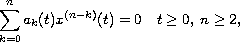where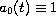,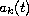are continuous bounded functions. Assuming that all the roots of the polynomial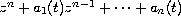are real and satisfy the inequality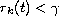for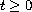and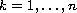, we prove that the solutions of the above equation satisfy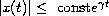for.

Submitted December 27, 2007. Published April 15, 2008.
Math Subject Classifications: 34A30, 34D20.
Key Words: Linear differential equations; Liapunov exponents; exponential stability.

Show me the PDF file (192 KB), TEX file, and other files for this article.Michael I. Gil' Department of Mathematics Ben Gurion University of the Negev P.0. Box 653, Beer-Sheva 84105, Israel Email: gilmi@cs.bgu.ac.il